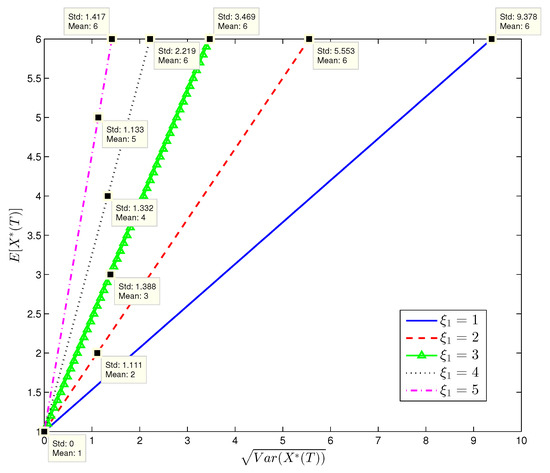Previous Article in Journal
Retiree Mortality Forecasting: A Partial Age-Range or a Full Age-Range Model?
Previous Article in Special Issue
Hedging with Liquidity Risk under CEV Diffusion
Open AccessArticle

# Effect of Variance Swap in Hedging Volatility Risk

byYang Shen
School of Risk and Actuarial Studies, University of New South Wales, Sydney, NSW 2052, Australia
Risks 2020, 8(3), 70; https://doi.org/10.3390/risks8030070
Received: 20 May 2020 / Revised: 18 June 2020 / Accepted: 23 June 2020 / Published: 1 July 2020
This paper studies the effect of variance swap in hedging volatility risk under the mean-variance criterion. We consider two mean-variance portfolio selection problems under Heston’s stochastic volatility model. In the first problem, the financial market is complete and contains three primitive assets: a bank account, a stock and a variance swap, where the variance swap can be used to hedge against the volatility risk. In the second problem, only the bank account and the stock can be traded in the market, which is incomplete since the idiosyncratic volatility risk is unhedgeable. Under an exponential integrability assumption, we use a linear-quadratic control approach in conjunction with backward stochastic differential equations to solve the two problems. Efficient portfolio strategies and efficient frontiers are derived in closed-form and represented in terms of the unique solutions to backward stochastic differential equations. Numerical examples are provided to compare the solutions to the two problems. It is found that adding the variance swap in the portfolio can remarkably reduce the portfolio risk. View Full-Text
Show FiguresFigure 1

MDPI and ACS Style

Shen, Y. Effect of Variance Swap in Hedging Volatility Risk. Risks 2020, 8, 70.